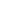Share

Hexadecimal is a base-16 number system. It is a different method of representing numbers than the base-10 system we use in every day practice. In base-10, we count in multiples of 10 before adding another digit. For example, "8 - 9 - 10 - 11 - 12..." and "98 - 99 - 100 - 101 - 102..." Notice how a new digit is added when the number 10 is reached, and another digit is added to represent 100 (10x10). In base-16, or the hexadecimal number system, each digit can have sixteen values instead of ten. The values of a hexadecimal digit can be:

0, 1, 2, 3, 4, 5, 6, 7, 8, 9, A, B, C, D, E, F

Therefore, the number 12 (in the common base-10 format) would be represented as "C" in hexadecimal notation. The number 24 would be 18 (16+8). 100 is 64 in hexadecimal (16x6 + 4) and 1000 is 3E8 (256x3 + 16x14 + 8).

While computers process numbers using the base-2, or binary system, it is often more efficient to visually represent the numbers in hexadecimal format. This is because it only takes one hexadecimal digit to represent four binary digits. Since there are eight binary digits in a byte, only two hexadecimal digits are needed to represent one byte.

Published: 2005

Definition from the PC Glossary